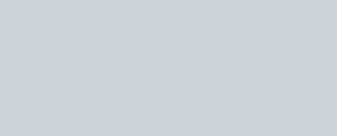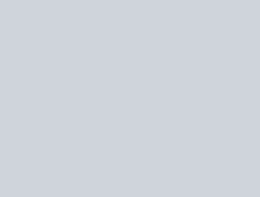Prove the following
Question:

If $\frac{d y}{d x}=y+3>0$ and $y(0)=2$, then $y(\ln 2)$ is equal to :-

1. 13

2. -2

3. 7

4. 5

Correct Option: , 3

Solution: